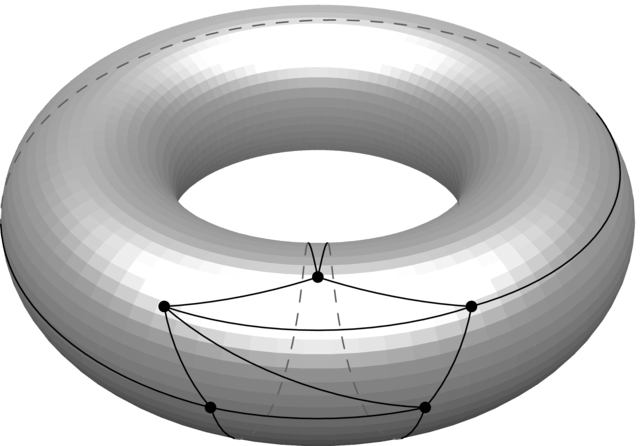# Chasing robbers on donuts

ICYMI. Enjoy! -AB

***

This week I discuss a question related to my research on graph theory. The question lies at the intersection of topology and graph searching games.

You might want to have a pad and paper for doodling nearby while you read. Or at least some donuts and coffee.

### Life on the surface of a donut

Life would seem mostly ordinary if you lived on the surface of the donut, as you could travel around in any direction and eventually come back to where you started. But there would be differences noticeable from life on the surface of a sphere (or imperfect oblate spheroid) like Earth. For one, if you made a lasso around the great equator, you couldn’t contract it to a point. A similar lasso around Earth’s equator would contract to either of the North or South Poles.

Another difference between spheres and donuts is the kinds of graphs you can draw on their surface. Planar graphs are those that can be drawn in the plane so their edges only touch at the nodes. For a more concrete idea, think of maps with dots at the capital cities and edges present if the countries share a border.

However, many graphs are not planar; for example, the complete graph on five nodes K5 isn’t planar. No matter how you try and draw it, at least one pair of edges overlap.

On a donut, however, you can draw K5 with no edge crossings. Seeing is believing.Drawing K5 on a torus with no edge crossings.

This kind of mathematical discussion takes us into the realm of topological graph theory, which studies graphs drawn on surfaces like spheres and donuts. There are different kinds of surfaces, but they all boil down to essentially a sphere with some holes (at least the orientable ones do and I’m not going to get into the non-orientable case here). The number of holes is the genus of a surface.

Graphs also have a genus. If a graph can be drawn without edge crossings on a surface with genus g (and nothing smaller than g), then the graph has genus g. For example, a planar graph has genus 0, while K5 has genus 1.

### Cops and Robbers

People find it funny when I tell them I play games in my mathematical research.

In the game of Cops and Robbers played on graphs, there is a bad guy (the robber) loose in a network that a group of good guys (the cops) are trying to capture. There are specified rules about how this can occur. The cops go first, choosing a set of nodes to occupy. The robber goes next and chooses a vertex, alternating moves with the cops at ticks of the clock. The players can only move from one node to another along edges; no teleporting allowed! The cops win if they can land on the node occupied by the robber. If the robber can avoid being captured forever, then the robber wins.

For example, consider a cycle with at least four nodes. You should convince yourself one cop can’t capture a robber, but two cops can do so.

Of course, we could place a cop at each node of our graph and win, but that is no fun. The cop number of a graph is the minimum number of cops we need to guarantee a win. So, the cop number of a cycle with at least four vertices is 2.

As an exercise, show that the cop number of the Petersen graph is 3.

Finding the cop number is not an easy problem and that can be made precise. It is NP-complete to compute the cop number on a general graph, meaning there is likely no efficient algorithm to solve it for all graphs. In a formal sense, Cops and Robbers is EXPTIME-complete which means it can be as complex as Chess played on an arbitrarily large, but finite, board.

We don’t even know how large the cop number can be. A graph is connected if every pair of nodes is joined by a path. Meyniel’s conjecture says the cop number of a connected graph is at most n1/2, where n is the number of nodes of the graph. We seem far from being able to prove Meyniel’s conjecture, and it is likely the toughest problem in this area of mathematics right now.

### Cops on donuts

An interesting question arises when we consider the cop number of graphs on surfaces. We know from early work by Aigner and Fromme in 1983 that planar graphs have cop number at most 3.

This is far from obvious! For example, how do three cops capture the robber on this graph?The Tutte graph has cop number 3.

Bernd Schroeder conjectured that the cop number of a graph with genus g is at most g +3. The conjecture holds for genus 0 graphs (that is, the planar ones), and he showed it holds for genus 1 graphs. In general,  the best upper bound we have for the cop number of genus g graphs is ⌊3/2g⌋ +3. Getting rid of the 3/2 seems to be a quite tough mathematical problem.r.

There you have it: we’ve arrived at a deep mathematical question about cops chasing robbers on donuts with many holes.

Isn’t mathematics infinitely amazing?

Anthony Bonato

## 2 thoughts on “Chasing robbers on donuts”

1.Toronto Scientific Research

Isn’t the #cops required just a variable of the maximum # of nodes surrounding any given node?

Like

1.abonato99

No. It’s more complicated than that.

Like